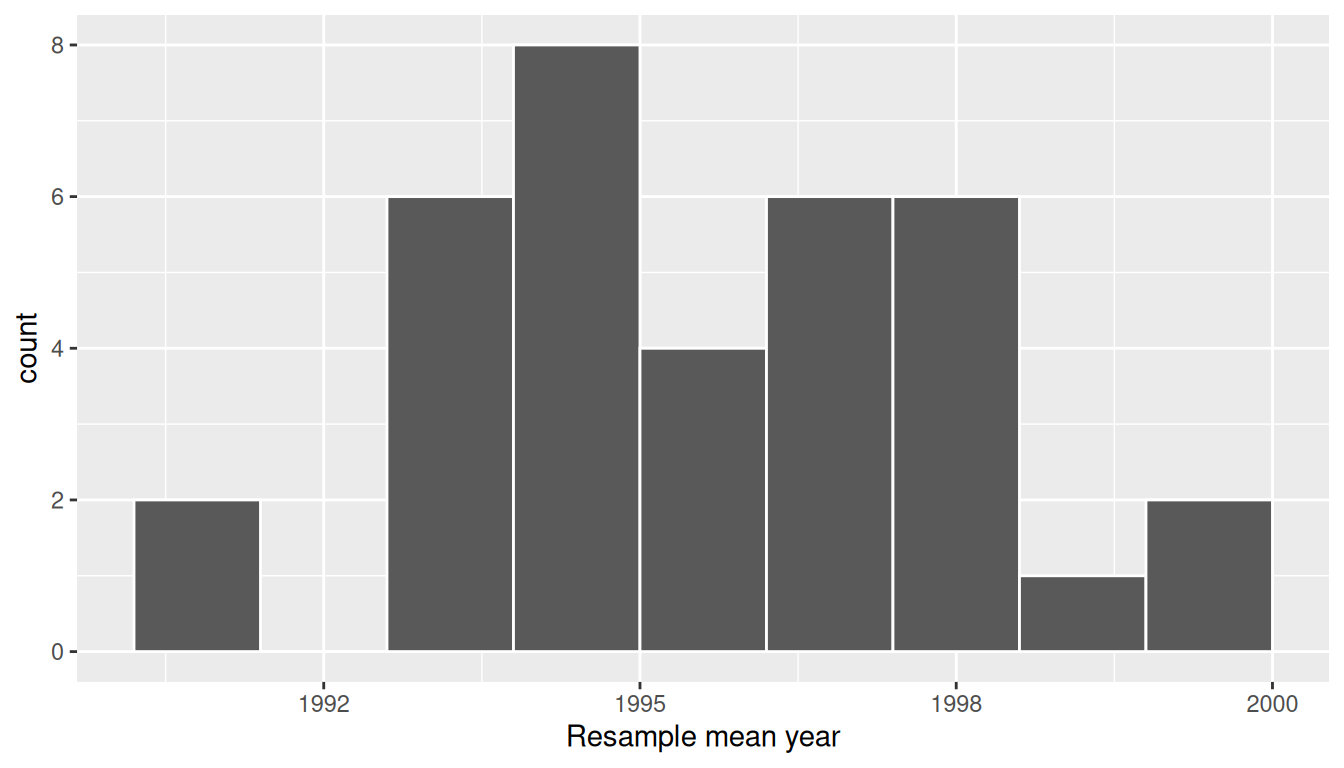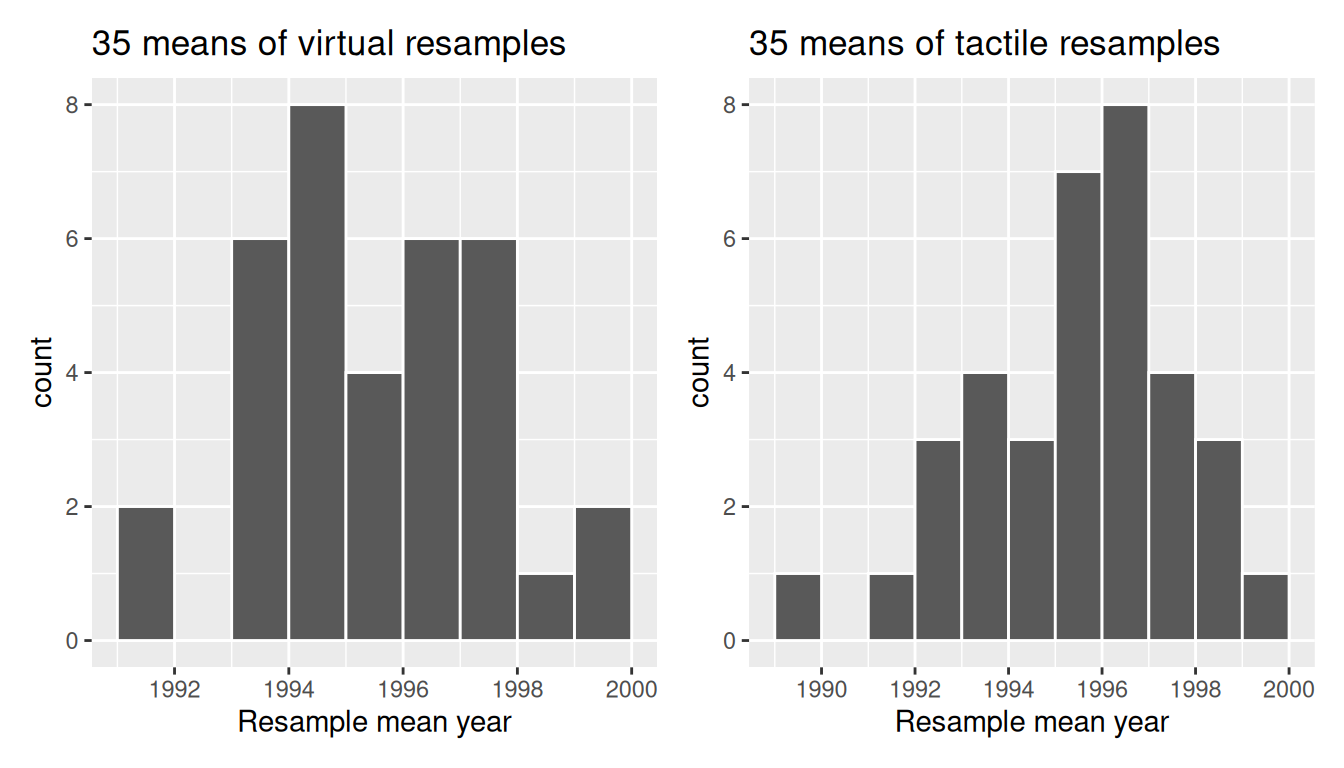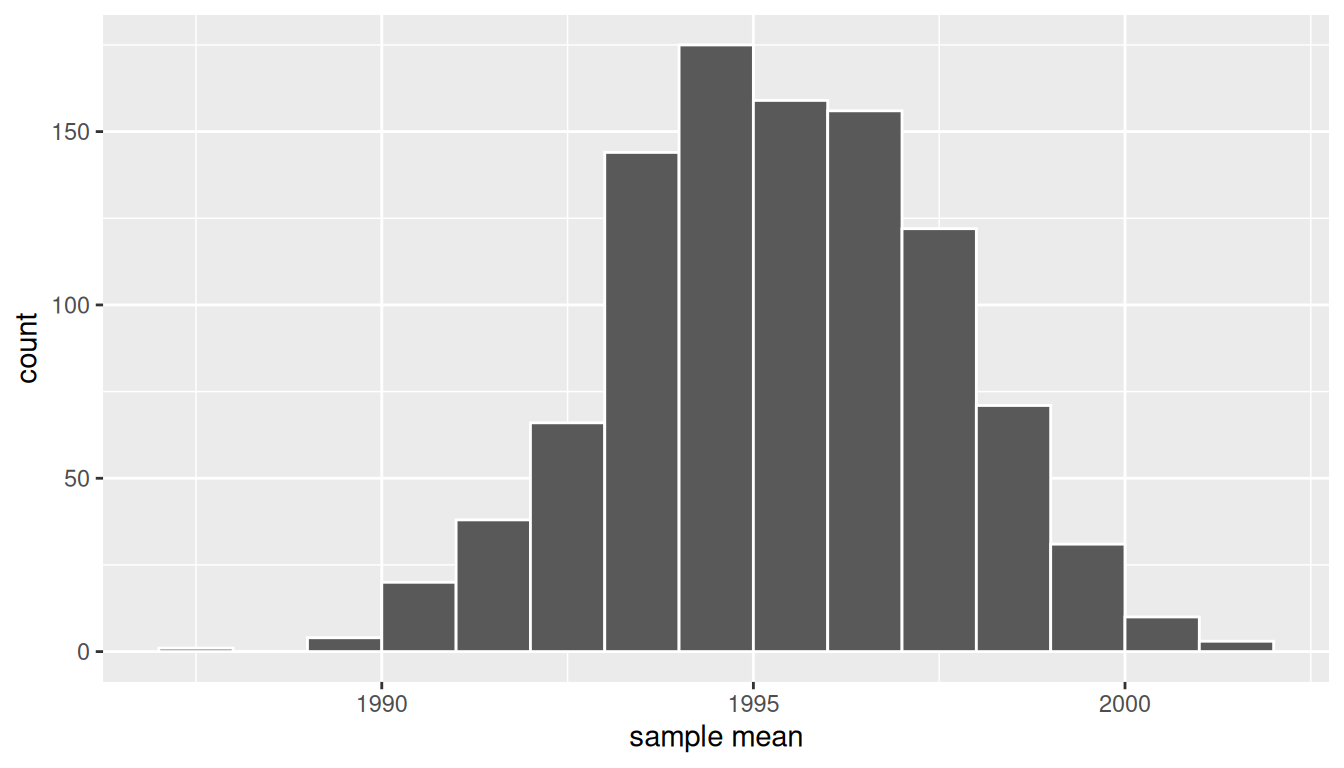## 8.2 Computer simulation of resampling

Let’s now mimic our tactile resampling activity virtually with a computer.

### 8.2.1 Virtually resampling once

First, let’s perform the virtual analog of resampling once. Recall that the pennies_sample data frame included in the moderndive package contains the years of our original sample of 50 pennies from the bank. Furthermore, recall in Chapter 7 on sampling that we used the rep_sample_n() function as a virtual shovel to sample balls from our virtual bowl of 2400 balls as follows:

virtual_shovel <- bowl %>%
rep_sample_n(size = 50)

Let’s modify this code to perform the resampling with replacement of the 50 slips of paper representing our original sample 50 pennies:

virtual_resample <- pennies_sample %>%
rep_sample_n(size = 50, replace = TRUE)

Observe how we explicitly set the replace argument to TRUE in order to tell rep_sample_n() that we would like to sample pennies with replacement. Had we not set replace = TRUE, the function would’ve assumed the default value of FALSE and hence done resampling without replacement. Additionally, since we didn’t specify the number of replicates via the reps argument, the function assumes the default of one replicate reps = 1. Lastly, observe also that the size argument is set to match the original sample size of 50 pennies.

Let’s look at only the first 10 out of 50 rows of virtual_resample:

virtual_resample
# A tibble: 50 x 3
# Groups:   replicate 
replicate    ID  year
<int> <int> <dbl>
1         1    37  1962
2         1     1  2002
3         1    45  1997
4         1    28  2006
5         1    50  2017
6         1    10  2000
7         1    16  2015
8         1    47  1982
9         1    23  1998
10         1    44  2015
# … with 40 more rows

The replicate variable only takes on the value of 1 corresponding to us only having reps = 1, the ID variable indicates which of the 50 pennies from pennies_sample was resampled, and year denotes the year of minting. Let’s now compute the mean year in our virtual resample of size 50 using data wrangling functions included in the dplyr package:

virtual_resample %>%
summarize(resample_mean = mean(year))
# A tibble: 1 x 2
replicate resample_mean
<int>         <dbl>
1         1          1996

As we saw when we did our tactile resampling exercise, the resulting mean year is different than the mean year of our 50 originally sampled pennies of 1995.44.

### 8.2.2 Virtually resampling 35 times

Let’s now perform the virtual analog of our 35 friends’ resampling. Using these results, we’ll be able to study the variability in the sample means from 35 resamples of size 50. Let’s first add a reps = 35 argument to rep_sample_n() to indicate we would like 35 replicates. Thus, we want to repeat the resampling with the replacement of 50 pennies 35 times.

virtual_resamples <- pennies_sample %>%
rep_sample_n(size = 50, replace = TRUE, reps = 35)
virtual_resamples
# A tibble: 1,750 x 3
# Groups:   replicate 
replicate    ID  year
<int> <int> <dbl>
1         1    21  1981
2         1    34  1985
3         1     4  1988
4         1    11  1994
5         1    26  1979
6         1     8  1996
7         1    19  1983
8         1    21  1981
9         1    49  2006
10         1     2  1986
# … with 1,740 more rows

The resulting virtual_resamples data frame has 35 $$\cdot$$ 50 = 1750 rows corresponding to 35 resamples of 50 pennies. Let’s now compute the resulting 35 sample means using the same dplyr code as we did in the previous section, but this time adding a group_by(replicate):

virtual_resampled_means <- virtual_resamples %>%
group_by(replicate) %>%
summarize(mean_year = mean(year))
virtual_resampled_means
# A tibble: 35 x 2
replicate mean_year
<int>     <dbl>
1         1   1995.58
2         2   1999.74
3         3   1993.7
4         4   1997.1
5         5   1999.42
6         6   1995.12
7         7   1994.94
8         8   1997.78
9         9   1991.26
10        10   1996.88
# … with 25 more rows

Observe that virtual_resampled_means has 35 rows, corresponding to the 35 resampled means. Furthermore, observe that the values of mean_year vary. Let’s visualize this variation using a histogram in Figure 8.12.

ggplot(virtual_resampled_means, aes(x = mean_year)) +
geom_histogram(binwidth = 1, color = "white", boundary = 1990) +
labs(x = "Resample mean year")FIGURE 8.12: Distribution of 35 sample means from 35 resamples.

Let’s compare our virtually constructed bootstrap distribution with the one our 35 friends constructed via our tactile resampling exercise in Figure 8.13. Observe how they are somewhat similar, but not identical.FIGURE 8.13: Comparing distributions of means from resamples.

Recall that in the “resampling with replacement” scenario we are illustrating here, both of these histograms have a special name: the bootstrap distribution of the sample mean. Furthermore, recall they are an approximation to the sampling distribution of the sample mean, a concept you saw in Chapter 7 on sampling. These distributions allow us to study the effect of sampling variation on our estimates of the true population mean, in this case the true mean year for all US pennies. However, unlike in Chapter 7 where we took multiple samples (something one would never do in practice), bootstrap distributions are constructed by taking multiple resamples from a single sample: in this case, the 50 original pennies from the bank.

### 8.2.3 Virtually resampling 1000 times

Remember that one of the goals of resampling with replacement is to construct the bootstrap distribution, which is an approximation of the sampling distribution. However, the bootstrap distribution in Figure 8.12 is based only on 35 resamples and hence looks a little coarse. Let’s increase the number of resamples to 1000, so that we can hopefully better see the shape and the variability between different resamples.

# Repeat resampling 1000 times
virtual_resamples <- pennies_sample %>%
rep_sample_n(size = 50, replace = TRUE, reps = 1000)

# Compute 1000 sample means
virtual_resampled_means <- virtual_resamples %>%
group_by(replicate) %>%
summarize(mean_year = mean(year))

However, in the interest of brevity, going forward let’s combine these two operations into a single chain of pipe (%>%) operators:

virtual_resampled_means <- pennies_sample %>%
rep_sample_n(size = 50, replace = TRUE, reps = 1000) %>%
group_by(replicate) %>%
summarize(mean_year = mean(year))
virtual_resampled_means
# A tibble: 1,000 x 2
replicate mean_year
<int>     <dbl>
1         1   1992.6
2         2   1994.78
3         3   1994.74
4         4   1997.88
5         5   1990
6         6   1999.48
7         7   1990.26
8         8   1993.2
9         9   1994.88
10        10   1996.3
# … with 990 more rows

In Figure 8.14 let’s visualize the bootstrap distribution of these 1000 means based on 1000 virtual resamples:

ggplot(virtual_resampled_means, aes(x = mean_year)) +
geom_histogram(binwidth = 1, color = "white", boundary = 1990) +
labs(x = "sample mean")FIGURE 8.14: Bootstrap resampling distribution based on 1000 resamples.

Note here that the bell shape is starting to become much more apparent. We now have a general sense for the range of values that the sample mean may take on. But where is this histogram centered? Let’s compute the mean of the 1000 resample means:

virtual_resampled_means %>%
summarize(mean_of_means = mean(mean_year))
# A tibble: 1 x 1
mean_of_means
<dbl>
1       1995.36

The mean of these 1000 means is 1995.36, which is quite close to the mean of our original sample of 50 pennies of 1995.44. This is the case since each of the 1000 resamples is based on the original sample of 50 pennies.

Congratulations! You’ve just constructed your first bootstrap distribution! In the next section, you’ll see how to use this bootstrap distribution to construct confidence intervals.

Learning check

(LC8.1) What is the chief difference between a bootstrap distribution and a sampling distribution?

(LC8.2) Looking at the bootstrap distribution for the sample mean in Figure 8.14, between what two values would you say most values lie?